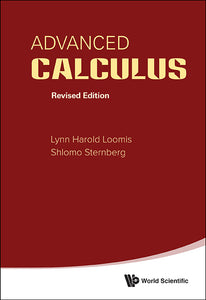Lynn Harold Loomis

Format: Print Book

ISBN: 9789814583923

• SGD 108.07
Unit price per
Tax included.

An authorised reissue of the long out of print classic textbook, Advanced Calculus by the late Dr Lynn Loomis and Dr Shlomo Sternberg both of Harvard University has been a revered but hard to find textbook for the advanced calculus course for decades.This book is based on an honors course in advanced calculus that the authors gave in the 1960's. The foundational material, presented in the unstarred sections of Chapters 1 through 11, was normally covered, but different applications of this basic material were stressed from year to year, and the book therefore contains more material than was covered in any one year. It can accordingly be used (with omissions) as a text for a year's course in advanced calculus, or as a text for a three-semester introduction to analysis.The prerequisites are a good grounding in the calculus of one variable from a mathematically rigorous point of view, together with some acquaintance with linear algebra. The reader should be familiar with limit and continuity type arguments and have a certain amount of mathematical sophistication. As possible introductory texts, we mention Differential and Integral Calculus by R Courant, Calculus by T Apostol, Calculus by M Spivak, and Pure Mathematics by G Hardy. The reader should also have some experience with partial derivatives.In overall plan the book divides roughly into a first half which develops the calculus (principally the differential calculus) in the setting of normed vector spaces, and a second half which deals with the calculus of differentiable manifolds.

Format: Hardcover
No of Pages: 596
Imprint: World Scientific
Publication date: 20140228